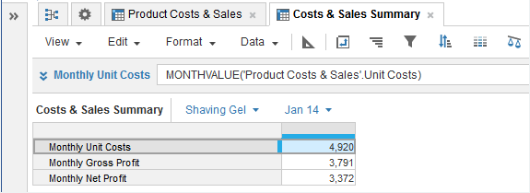1. Calculation functions
2. All Functions
3. Time and Date Functions
4. MONTHVALUEReturns the summary month value of the source line item or property that matches the time summary setting for the source.

## Syntax

`MONTHVALUE(x) `

where:

• x: Line item or property to be summarized.

## Format

Input Format Output Format

x: Line item - number, Boolean, date, time period, list, text.

Matches the input format.

## Arguments

The function uses the following arguments:

• x: Number, Boolean, date, time period, list item, text.

## Constraints

The function has the following constraints:

• The result formatting must match the source formatting.
• The result must have a timescale applied.
• The time period granularity of the source should be Month, Week, or Day. If you make the source time period granularity Quarter, for example, MONTHVALUE returns zero.
• If the time summary method is None, only numbers get values. Use other time summary methods to get values for Booleans, dates, time periods, list items, and text. See Summary Methods and Time Aggregation for more information.

## Excel equivalent

• No Excel equivalent

## Examples

### Sum example

In this example, the time summary method for the Net Profit number-formatted source line item is Sum: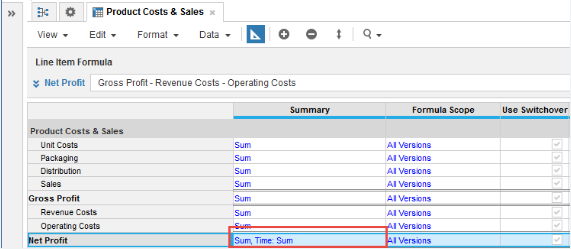The Month Net Profit result line item uses the formula below to return the summed value for each month period in the timescale:

`MONTHVALUE(Net Profit)        `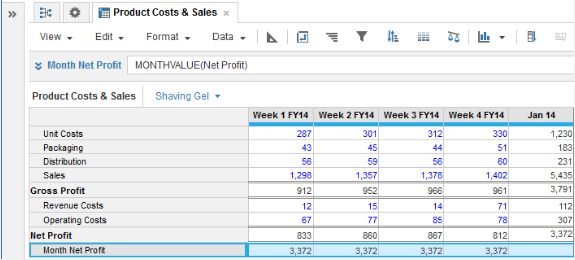### Average example

The time summary method for the Net Profit line item is set to Average: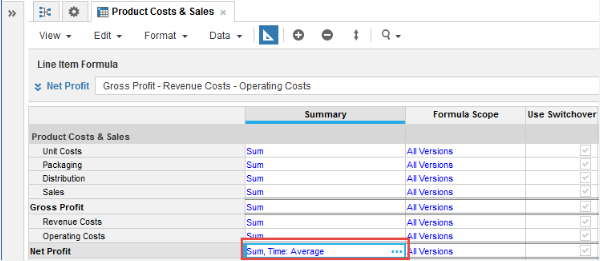Net Profit now displays the average weekly value for each month period:

`MONTHVALUE(Net Profit)`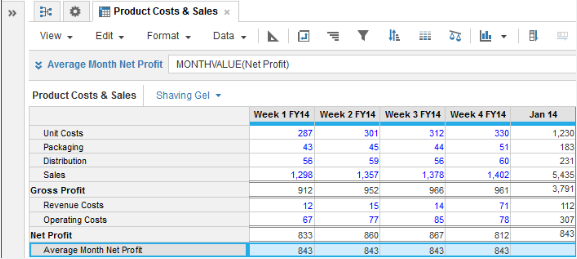### Max example

The time summary method for the Net Profit line item is set to Max: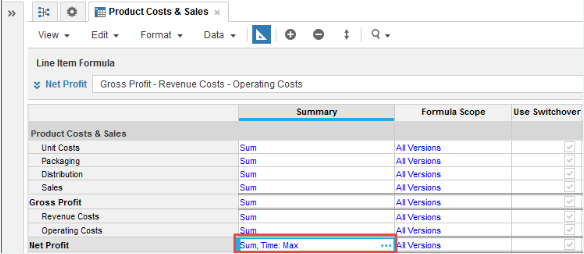Maximum Month Net Profit returns the maximum weekly value for each month period:

`MONTHVALUE(Net Profit)`

### Closing Balance example

In this example, Product Costs & Sales is the source module for unit costs and for gross and net profit figures for main products.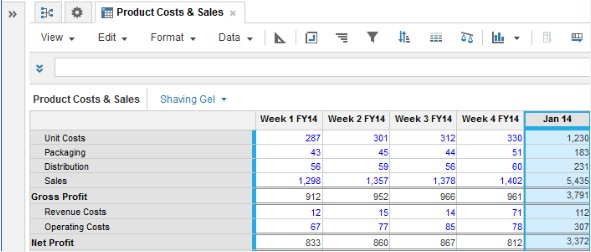The time summary method is set to Sum for Unit Costs, Gross Profit, and Net Profit source line items.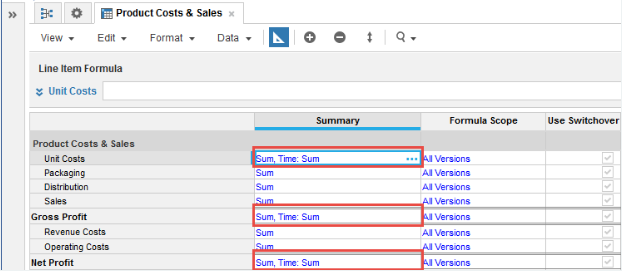In a Costs & Sales Summary results module with a timescale applied, use MONTHVALUE to return month summary values for Monthly Unit Costs, Monthly Gross Profit, and Monthly Net Profit:

```MONTHVALUE('Product Costs & Sales'.Unit Costs)
```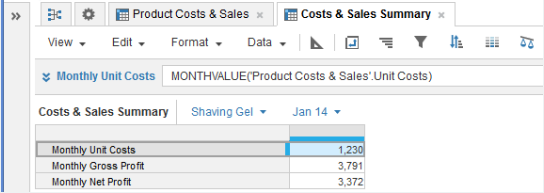In this example, ensure that the time summary method for the results line items gives you the values that you want.

If you change the time summary method for Monthly Unit Costs from Closing Balance to Sumthe value returned for the Jan 14 selection where the result line item timescale is set to Weeks, is incorrect.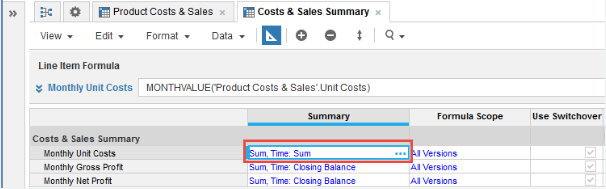This result happens because the week value is multiplied to summarize for the month:

4 x 1,230 = 4,920